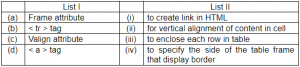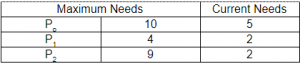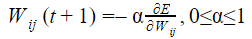## UGC-NET DEC-2019 Part-2

 Question 1
Consider the following statements:
(a) The running time of dynamic programming algorithm is always θ(ρ) where ρ is number of subproblems.
(b) When a recurrence relation has cyclic dependency, it is impossible to use that recurrence relation (unmodified) in a correct dynamic program.
(c) For a dynamic programming algorithm, computing all values in a bottom-up fashion is asymptotically faster than using recursion and memorization.
(d) If a problem X can be reduced to a known NP-hard problem, then X must be NP-hard.
Which of the statement(s) is (are) true?
 A Only (b) and (a) B Only (b) C Only (b) and (c) D Only (b) and (d)
Algorithms       Dynamic-Programming
Question 1 Explanation:
(i). FALSE: The running time of a dynamic program is the number of subproblems times the time per subproblem. This would only be true if the time per subproblem is O(1).
(ii). TRUE: Cyclic dependency is a relation between two or more modules which either directly or indirectly depend on each other to function properly. Such modules are also known as mutually recursive. So, we can’t get correct solution when we are using dynamic programming.
(iii). FALSE: A bottom up implementation must go through all of the sub-problems and spend the time per subproblem for each. Using recursion and memoization only spends time on the subproblems that it needs. In fact, the reverse may be true: using recursion and memoization may be asymptotically faster then a bottom-up implementation.
(iv). FALSE: If a problem X can be reduced to a known NP-hard problem, then X must be NP-Complete.
 Question 2
Let G = (V, T, S, P) be any context-free grammar without any λ-productions or unit productions. Let K be the maximum number of symbols on the right of any production P. The maximum number of production rules for any equivalent grammar in Chomsky normal form is given by:
 A (K – 1) |P| + |T| - 1 B (K – 1) |P| +| T| C K |P| + |T| - 1 D K |P| + |T|
Theory-of-Computation       Context-Free-Grammar
Question 2 Explanation:
Let G = (V, T, S, P) be any context-free grammar without any λ-productions or unit productions. Let K be the maximum number of symbols on the right of any production P. The maximum number of production rules for any equivalent grammar in Chomsky normal form is given by (K – 1) |P| + |T|
 Question 3
Which of the following is not needed by an encryption algorithm used in Cryptography?
 A KEY B Message C Ciphertext D User details E Option-C and Option-D
Computer-Networks       Network-Security
Question 3 Explanation:
To Encrypt or decrypt an algorithm it requires Plain Text(or Message) and Key(either public or private).
But it does not require User details.
Not relevant option-"cipher text". Note: According to final answer key, given marks to option-C and Option-D
 Question 4
A Non-pipelined system takes 30ns to process a task. The same task can be processed in a four-segment pipeline with a clock cycle of 10ns. Determine the speed up of the pipeline for 100 tasks
 A 3 B 4 C 3.91 D 2.91
Computer-Organization       Pipelining
Question 4 Explanation:
S=ntn / (n+k-1)tp
= 100*30/(100+4-1)*10
= 3000 / 1030
= 2.91
 Question 5
Which tag is used to enclose any number of javascript statements in HTML document?
 A < code > B < script > C < title > D < body >
Web-Technologies       HTML
Question 5 Explanation:
The < script > tag is used to define a client-side script (JavaScript).
The < script > element either contains scripting statements, or it points to an external script file through the src attribute.
Common uses for JavaScript are image manipulation, form validation, and dynamic changes of content.
 Question 6
Consider the following languages: L1 = {anbncm} ∪ {an bm cm}, n. m ≥ o L2 = {ωωR |ω ∈ {a, b}*} Where R represents reversible operation. Which one of the following is (are) inherently ambiguous language(s)?
 A only L1 B only L2 C both L1 and L2 D neither L1 nor L2
Theory-of-Computation       Context-Free-Language
Question 6 Explanation:
A language is inherently ambiguous:
If for a language every existing grammar is ambiguous then that language is called inherently ambiguous language. By this definition of inherently ambiguous, we can conclude that for an inherently ambiguous language an equivalent unambiguous grammar can't be found.
There are several languages (special types ) which are inherently ambiguous.
For example : L= {ai bj ck | i=j or c=k} is inherently ambiguous
The reason is : It is union of two languages {an bn cm} U {an bm cm}
So it will have grammar
S-> A | B
A-> PQ
P->aPb | ab
Q-> cQ | c
B-> UV
U-> aU | a
V-> bVc | bc
Grammar A generates equal number of a's and b's and any number of c's
Grammar B generates equal number of b's and c's and any number of a's
So the strings which have equal numbers of a's, b's and c's can be generated by A or B
So for these strings we always have two parse trees.
Hence this language is ambiguous as we cannot make an unambiguous grammar for it.
 Question 7
Match List-I with List-II:Choose the correct option from those given below:
 A (a).(i), (b).(ii), (c).(iii), (d).(iv) B (a).(ii), (b).(i), (c).(iii), (d).(iv) C (a).(iv), (b).(iii), (c).(ii), (d).(i) D (a).(iii), (b).(iv), (c).(ii), (d).(i)
Web-Technologies       HTML
Question 7 Explanation:
Frame attribute → To specify the side of the table frame that display border
The < frame > tag defines one particular window (frame) within a < frameset >.
Each < frame > in a < frameset > can have different attributes, such as border, scrolling, the ability to resize, etc.
< tr > tag → To enclose each row in a table.
The < tr > tag defines a row in an HTML table.
A < tr > element contains one or more or elements
Valign attribute → for vertical alignment of content in cell
< a > tag → To create link in HTML
 Question 8
A network with a bandwidth of 10 Mbps can pass only an average of 12,000 frames per minute with each frame carrying an average of 10,000 bits. What is the throughput of this network?
 A 1,000,000 bps B 2,000,000 bps C 12,000,000 bps D 1,200,00,000 bps
Computer-Networks       Throughput
Question 8 Explanation:
Given data,
Bandwidth= 10 Mbps
Frames per minute=12000
Each frame per bits=10000
Throughput=?
Step-1: Here, they given each frame per minute. So, convert into seconds is
(12000*10000) / 60
Step-2: 120000000 / 60
= 2000000
It is nothing but 2Mbps
 Question 9If L is the regular language denoted by T= (w + x*)(ww)*, then the regular language h(L) is given by
 A (z x yy + xy) (z x yy) B (zxyy + (xzy)*) (zxyy zxyy)* C (zxyy + xyz) (zxyy)* D (zxyy + (xzy)* (zxyy zxyy)
Theory-of-Computation       Regular-Language
 Question 10
A tree has 2n vertices of degree 1, 3n vertices of degree 2, n vertices of degree 3. Determine the number of vertices and edges in tree.
 A 12.11 B 11.12 C 10.11 D 9.1
Engineering-Mathematics       Graph-Theory
 Question 11

Given CPU time slice of 2ms and following list of processes.Find average turnaround time and average waiting time using round robin CPU scheduling?
 A 4.0 B 5.66, 1.66 C 5.66, 0 D 7, 2
Operating-Systems       CPU-Scheduling
Question 11 Explanation:Average Turnaround Time= (5+5+7)/3
= 5.66
Average Waiting Time= (2+1+2)/3
= 1.66

 Question 12
Consider the following statements:
S1: If a group (G,*) is of order n, and a ∈ G is such that am = e for some integer m ≤ n, then m must divide n.
S2: If a group (G,*) is of even order , then there must be an element and a ∈ G is such that a ≠ e and a * a = e.
Which of the statements is (are) correct?
 A Only S1 B Only S2 C Both S1 and S2 D Neither S1 nor S2
Engineering-Mathematics       Set-Theory
 Question 13
What is the worst case running time of Insert and Extract-min, in an implementation of a priority queue using an unsorted array? Assume that all insertions can be accommodated.
 A θ(1) , θ(n) B θ(n) , θ(1) C θ(1) , θ(1) D θ(n) , θ(n)
Data-Structures       Queues-and-Stacks
Question 13 Explanation:
Insertion will remain the same for best,average and worst case time complexities. It will take a constant amount of time only.
Extract-Min will take θ(n) time for above conditions. The array is unsorted there is naturally no better algorithm than just linear search. Worst case performance for linear search is Θ(n)
 Question 14
Consider the following statements: S1: These exists no algorithm for deciding if any two Turning machine M1 and M2 accept the same language. S2: Let M1 and M2 be arbitrary Turing machines. The problem to determine L(M1)⊆ L(M2)is undecidable. Which of the statements is (are) correct?
 A Only S1 B Only S2 C Both S1 and S2 D Neither S1 nor S1
Theory-of-Computation       Turing-machines
Question 14 Explanation:
Statement S1 is correct because equality problem of recursively enumerable languages is undecidable.
Statement S2 is correct because subset problem of recursively enumerable languages is undecidable.
 Question 15
Suppose a system has 12 magnetic tape drives and at time to, three processes are allotted tape drives out of their need as given below:At time to, the system is in safe state. Which of the following is safe sequence so that deadlock is avoided?
 A (p0, p1, p2) B (p1, p0, p2) C (p2, p1, p0) D (p0, p2, p1)
Question 15 Explanation:Out of Total 12 resources, 9 resources are allocated. So the remaining resources are 3. With 3 resources process P1 requirements can be fulfilled. So after that total resources will be 3+ resources allocated to P1(i.e. 3+2= 5)
Now process P0 requirement will be fulfilled. So after that total resources will be 5+ resources allocated to P0(i.e. 5+5=10)
Now process P2 requirement will be fulfilled. So after that total resources will be 10+ resources allocated to P2(i.e. 10+2=12)
 Question 16

Let Wo represents weight between node i at layer k and node j at layer (k – 1) of a given multilayer perceptron. The weight updation using gradient descent method is given by

Where α and E represents learning rate and Error in the output respectively?
 ABCDQuestion 17
The reduced Instruction Set Computer (RISC) characteristics are:
(a) Single cycle instruction execution
(b) Variable length instruction formats
(c) Instructions that manipulates operands in memory
(d) Efficient instruction pipeline
Choose the correct characteristics from the options given below:
 A (a) and (b) B (b) and (c) C (a) and (d) D (c) and (d)
Computer-Organization       RISC-and-CISC
Question 17 Explanation:
Common RISC characteristics
→ Load/store architecture (also called register-register or RR architecture) which fetches operands and results indirectly from main memory through a lot of scalar registers. Other architecture is storage-storage or SS in which source operands and final results are retrieved directly from memory.
→ Fixed length instructions which (a) are easier to decode than variable length instructions, and (b) use fast, inexpensive memory to execute a larger piece of code. → Hardwired controller instructions (as opposed to microcoded instructions). This is where RISC really shines as hardware implementation of instructions is much faster and uses less silicon real estate than a microstore area.
→ Fused or compound instructions which are heavily optimized for the most commonly used functions.
→ Pipelined implementations with the goal of executing one instruction (or more) per machine cycle.
→ Large uniform register set
→ Minimal number of addressing modes
→ no/minimal support for misaligned accesses
Characteristic of CISC:
→ Complex instruction, hence complex instruction decoding.
→ Instruction are larger than one word size.
→ Instruction may take more than a single clock cycle to get executed.
→ Less number of general purpose register as operation get performed in memory itself.
→ More Data types.
 Question 18
A microinstruction format has microoperation field which is divided into 2 subfields F1 and F2. Each having 15 distinct micro operations condition field CD for four status bits. Branch field BR having four options used in conjunction with address field AD. The address space is of 128 memory words. The size of micro instruction is
 A 19 B 18 C 17 D 20
Computer-Organization       Microprogrammed-Control-Unit
 Question 19
B-Tree, each node represents a disk block. Suppose one block holds 8192 bytes. Each key uses 32 bytes. In a B-tree of order M there are M – 1 keys. Since each branch is on another disk block, we assume a branch is of 4 bytes. The total memory requirement for a non-leaf node is
 A 32 M – 32 B 36 M – 32 C 36 M – 36 D 32 M – 36
Database-Management-System       B-and-B+-Trees
Question 19 Explanation:
The size of non-leaf node in B-tree = m(Pb) + (m-1)(key+ Pr)
Where Pb is Block pointer and Pr is record pointer.
In question,Pb = 4 and key size = 32 and since the size of Pr is not given in question consider it as zero.
Hence size of non-leaf node in B-tree = m(4) + (m-1)(32)
= 36M - 32
 Question 20
Which of the component module of DBMS does rearrangement and possible ordering of operations, eliminate redundancy in query and use efficient algorithms and indexes during the execution of a query?
 A query compiler B query optimizer C Stored data manager D Database processor
Database-Management-System       Relational-databases
Question 20 Explanation:
The query optimizer (called simply the optimizer) is built-in database software that determines the most efficient method for a SQL statement to access requested data. The optimizer choose the plan with the lowest cost among all considered candidate plans(the cost computation accounts for factors of query execution such as I/O, CPU, and communication).
It rearrange and does the possible ordering of operations, eliminate redundancy in query and use efficient algorithms and indexes during the execution of a query
 Question 21
Consider the following grammar :
S→0A|0BB
A→00A| λ
B→1B|11C
C→B
Which language does this grammar generate?
 A L(00) * 0+(11)*1) B L(0(11)* + 1(00)*) C L((00)*0) D L(0(11) *1)
Theory-of-Computation       Languages-and-Grammars
Question 21 Explanation:
Option 1 is incorrect because every string generated by given grammar will contain only 0's and given option is generating string containing 1's also. Option 2 is incorrect because it can't generate "000". Option 3 is correct because it generates string same as given grammar do. Option 4 is incorrect because every string generated by given grammar will contain only 0's and given option is generating string containing 1's also.
 Question 22
Consider the following Linear programming problem (LPP) :
Maximize z = x1 + x2
Subject to the constraints:The solution of the above LPP is:
 A x1=750, x2=750, z=1500 B x1=500, x2=100, z=1500 C x1=1000, x2=500, z=1500 D x1=900, x2=600, z=1500
Engineering-Mathematics       Combinatorics
 Question 23
Let A be the base class in C++ and B be the derived class from A with protected inheritance.
Which of the following statement is false for class B?
 A Member function of class B can access protected data of class A B Member function of class access public data of class A C Member function of class B cannot access private data of class A D Object of derived class B can access public base class data
OOPS       Inheritance
Question 23 Explanation:
A→ Base class
B→ Derived class from A with protected inheritance.
The friend functions and the member functions of a friend class can have direct access to both the PRIVATE and PROTECTED data, the member functions of a derived class can directly access only the PROTECTED data.
However, they can access the PRIVATE data through the member functions of the base class TRUE: Member function of class B can access protected data of class A
TRUE: Member function of class access public data of class A
FALSE: Member function of class B cannot access private data of class A
TRUE: Object of derived class B can access public base class data
 Question 24
Given two tables EMPLOYEE (EID, ENAME, DEPTNO)
DEPARTMENT (DEPTNO. DEPTNAME)
Find the most appropriate statement of the given query:
Select count (*) ‘total’
from EMPLOYEE
where DEPTNO IN (D1, D2)
group by DEPTNO
having count (*) > 5
 A Total number of employees in each department D1 and D2 B Total number of employees of department D1 and D2 if their total is >5 C Display total number of employees in both departments D1 and D2 D The output of the query must have atleast two rows
Database-Management-System       SQL
 Question 25
Which of the following are legal statements in C programming language?
(a) int *P = &44;
(b) int *P = &r;
(c) int P = &a;
(d) int P = a:
Choose the correct option:
 A (a) and (b) B (b) and (c) C (b) and (d) D (a) and (d)
Programming       C-Programming
Question 25 Explanation:
Legal Statements:
int *P = &r;
int P = a:
Illegal Statements:
int *P = &44;
int P= &a;
There are 25 questions to complete.

Register Now